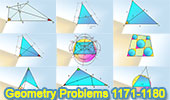# Geometry Problems 1171-1180. Level: High School, CollegeGeometry Problems 1171 - 1180Geometry Problem 1171 Area of a Triangle in terms of the three altitude or heights ha, hb, hc. Geometry Problem 1172 Cyclic Quadrilateral, Circle, Secant, Triangle, Similarity, Metric Relations. Geometry Problem 1173 Triangle, Cevian, Angle, Congruence, Similarity. Geometry Problem 1174 Triangle, Quadrilateral, Double, Triple, Angle, Congruence, Excenter, Angle Bisector. Geometry Problem 1176 Cyclic Quadrilateral, Diagonals, Six Diameters, Circles, Collinear Points, Concurrent Lines. Geometry Problem 1177 Triangle, Two Angle Bisectors, Metric Relations. Geometry Problem 1178 Triangle, Angle Bisector, Metric Relations. Geometry Problem 1179 Triangle, Centroid, Transversal, Sum of Ratios, Sides. Geometry Problem 1180 Quadrilateral, 120 Degrees, Diagonals, Perpendicular.

Home | Sitemap | Search | Geometry | Problems | Visual Index | 10 ProblemsEmail | Post a comment | by Antonio Gutierrez
Last updated: Mar 4, 2018